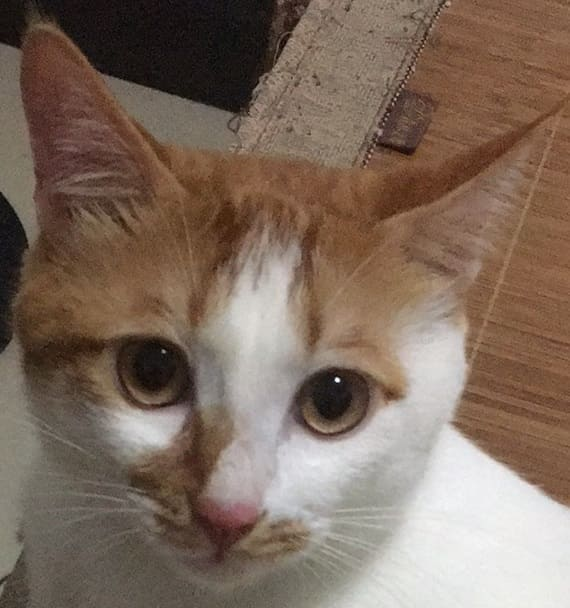C++11智能指针的使用过程中，一个很大的陷阱就是陷入了循环引用问题，这个问题类似于大型项目中两个头文件互相包含的问题，而在C++11中如果因为使用智能指针而引发了循环引用问题，那么其结果会导致智能指针无法释放，导致动态内存泄漏。

# 1 循环引用

class B;
class A {
public:
std::shared_ptr<B> pointer_B;
~A() {
std::cout << "A已经被删除" << std::endl;
}
};

class B {
public:
std::shared_ptr<A> pointer_A;
~B() {
std::cout << "B已经被删除" << std::endl;
}

};

int main()
{
{
std::shared_ptr<A> pointer_A(new A);
std::shared_ptr<B> pointer_B(new B);
pointer_A->pointer_B = pointer_B;
pointer_B->pointer_A = pointer_A;
}

getchar();
return 0;
}



# 2 使用weak_ptr修复循环引用

class B;
class A {
public:
std::shared_ptr<B> pointer_B;
~A() {
std::cout << "A已经被删除" << std::endl;
}
};

class B {
public:
std::weak_ptr<A> pointer_A;// 将shared_ptr修改为weak_ptr
~B() {
std::cout << "B已经被删除" << std::endl;
}

};

int main()
{
{
std::shared_ptr<A> pointer_A(new A);
std::shared_ptr<B> pointer_B(new B);
pointer_A->pointer_B = pointer_B;
pointer_B->pointer_A = pointer_A;
}

getchar();
return 0;
}支付宝扫一扫微信扫一扫纵使晴明无雨色，入云深处亦沾衣。